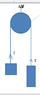# Friction between pulley and rope

avast2
I have a question about friction between pulley and rope?
Does anyone know how to calculate friction between pulley and rope
I enclosed an example picture
Thank you!

#### Attachments

•Untitled.png
2.8 KB · Views: 114

I have a question about friction between pulley and rope?
Does anyone know how to calculate friction between pulley and rope
I enclosed an example picture
Thank you!
If the tensions are the same on both sides, the net friction is zero.

•davenn
DrStupid
If the tensions are the same on both sides, the net friction is zero.

You may assume zero friction in theory but in reality there is at least static friction. With equal tension all around the pulley I would expect it to be

##F_f \le \frac{\pi }{2} \cdot \mu \cdot F_0##

where ##F_0## is the total force acting on the pulley.

Last edited:
You may assume zero friction in theory but in reality there is at least static friction. With equal tension all around the pulley I would expect it to be

##F_f \le \frac{\pi }{2} \cdot \mu \cdot F_0##

where ##F_0## is the total force acting on the pulley.
I was assuming a massless rope.

Homework Helper
Gold Member
I have a question about friction between pulley and rope?
Does anyone know how to calculate friction between pulley and rope
https://en.m.wikipedia.org/wiki/Capstan_equation

If the rope fits too tight inside the pulley's groove, friction should be greater than calculated by above equation due to wedge effect.

Last edited:
•jrmichler and DrStupid
DrStupid
I was assuming a massless rope.

The mass of the rope is not the problem. It just changes the total force and can be neglected in the situation discussed. I started the derivation for the case that the tension can not assumed to be constant and already realized that the force increases exponentially. Thaks @Lnewqban's link to the Capstan equation I don't need to finish my calculation.

•Lnewqban
Gold Member
From school boy memories, I believe the frictional resistance for a rope wrapped round an object is the tension multiplied by e^(mu theta).

•